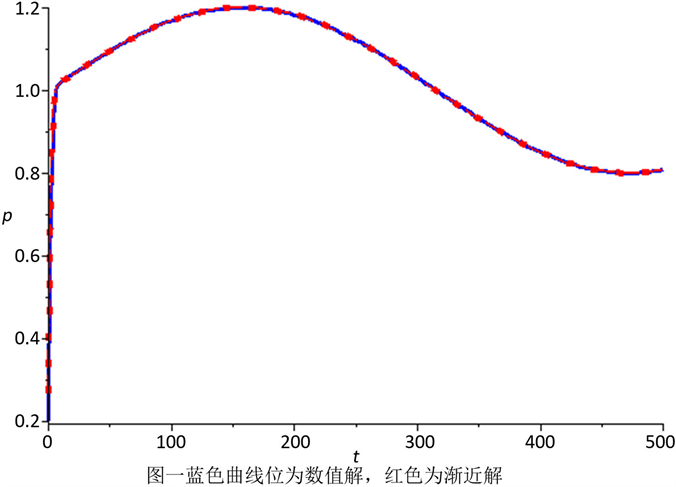# 关于时间慢变的广义Logistic模型的多尺度分析Multiscale Analysis of a Generalized Logistic Model with Slowly Varying Parameters

• 全文下载: PDF(664KB)    PP.956-961   DOI: 10.12677/AAM.2018.77112
• 下载量: 299  浏览量: 1,268

In the generalized logistic model, there are three parameters, R (intrinsic rate of growth), K (maxi-mum tolerance of population) and β (the degree of population using environmental resources), which are constants in the model. In fact, they vary slowly with time in the natural environment. It is hard to obtain the exact solution. In this paper we get the asymptotic solution of the model using the method of multi-scale. Numerical simulation illustrates the validity of the asymptotic solution obtained.

1. 引言

$\frac{\text{d}M}{M\text{d}T}=R\left(\frac{K-M}{K+\left(R/C\right)M}\right),$ (1)

$\frac{\text{d}X}{\text{d}T}=\frac{R\left(T\right)X\left(1-\frac{X}{K\left(T\right)}\right)}{1+\epsilon \stackrel{¯}{\beta }\left(T\right)X},\text{\hspace{0.17em}}\text{\hspace{0.17em}}X\left(0\right)={X}_{0}.$ (2)

$K\left(\epsilon t\right)={K}_{0}k\left(\epsilon t\right),\text{\hspace{0.17em}}\stackrel{¯}{\beta }\left(\epsilon t\right)={\beta }_{0}\beta \left(\epsilon t\right),\text{\hspace{0.17em}}R\left(\epsilon t\right)={R}_{0}r\left(\epsilon t\right),$ (3)

(2)式变换为

$\frac{\text{d}x\left(t,\epsilon \right)}{\text{d}t}=\frac{r\left(\epsilon t\right)x\left(1-\frac{x\left(t,\epsilon \right)}{k\left(\epsilon t\right)}\right)}{1+\epsilon \sigma \beta \left(\epsilon t\right)x\left(t,\epsilon \right)},\text{\hspace{0.17em}}x\left(0,\epsilon \right)=\mu .$ (4)

2. 多尺度分析

${t}_{0}=\frac{1}{\epsilon }g\left({t}_{1}\right),\text{\hspace{0.17em}}\text{\hspace{0.17em}}{t}_{1}=\epsilon t,$ (5)

${t}_{1}$ 相对于 ${t}_{0}$ 为慢时间尺度，且需满足 $g\left({t}_{1}\right)>0\left({t}_{1}>0\right),\text{\hspace{0.17em}}g\left(0\right)=0$ ，这里 $r,k,\stackrel{¯}{\beta }$ 都变为关于 ${t}_{\text{1}}$ 的函数 $r\left({t}_{1}\right),k\left({t}_{1}\right),\stackrel{¯}{\beta }\left({t}_{1}\right)$ 。此外，为了让 ${t}_{0}$${t}_{1}$ 一一对应，我们需要假定 ${g}^{\prime }\left({t}_{1}\right)>0$

$x\left(t,\epsilon \right)$ 看作两个时间尺度的函数 $x\left({t}_{\text{0}},{t}_{1},\epsilon \right)$ ，再运用链式法则得到的多尺度等式

${g}^{\prime }\left({t}_{1}\right){D}_{\text{0}}x\left(t,\epsilon \right)+\epsilon {D}_{\text{1}}x\left(t,\epsilon \right)=\frac{r\left(\epsilon t\right)x\left(1-\frac{x\left(t,\epsilon \right)}{k\left(\epsilon t\right)}\right)}{1+\epsilon \sigma \beta \left(\epsilon t\right)x\left(t,\epsilon \right)},$ (6)

$x\left({t}_{\text{0}},{t}_{1},\epsilon \right)={x}_{0}\left({t}_{0},{t}_{1}\right)+\epsilon {x}_{1}\left({t}_{0},{t}_{1}\right)+{\epsilon }^{2}{x}_{2}\left({t}_{0},{t}_{1}\right)+\cdots ,$ (7)

$\begin{array}{l}{g}^{\prime }\left({t}_{1}\right){D}_{0}\left[{x}_{0}\left({t}_{0},{t}_{1}\right)+\epsilon {x}_{1}\left({t}_{0},{t}_{1}\right)+{\epsilon }^{2}{x}_{2}\left({t}_{0},{t}_{1}\right)+\cdots \right]+\epsilon {D}_{1}\left[{x}_{0}\left({t}_{0},{t}_{1}\right)+\epsilon {x}_{1}\left({t}_{0},{t}_{1}\right)+{\epsilon }^{2}{x}_{2}\left({t}_{0},{t}_{1}\right)+\cdots \right]\\ =\frac{r\left(\epsilon t\right)\left({x}_{0}\left({t}_{0},{t}_{1}\right)+\epsilon {x}_{1}\left({t}_{0},{t}_{1}\right)+{\epsilon }^{2}{x}_{2}\left({t}_{0},{t}_{1}\right)+\cdots \right)\left(1-\frac{{x}_{0}\left({t}_{0},{t}_{1}\right)+\epsilon {x}_{1}\left({t}_{0},{t}_{1}\right)+{\epsilon }^{2}{x}_{2}\left({t}_{0},{t}_{1}\right)+\cdots }{k\left(\epsilon t\right)}\right)}{1+\epsilon \sigma \beta \left(\epsilon t\right)\left({x}_{0}\left({t}_{0},{t}_{1}\right)+\epsilon {x}_{1}\left({t}_{0},{t}_{1}\right)+{\epsilon }^{2}{x}_{2}\left({t}_{0},{t}_{1}\right)+\cdots \right)}.\end{array}$ (8)

${g}^{\prime }\left({t}_{1}\right){D}_{0}{x}_{0}=r\left({t}_{1}\right){x}_{0}\left(1-\frac{{x}_{0}}{k\left({t}_{1}\right)}\right);$ (9)

${g}^{\prime }\left({t}_{1}\right){D}_{0}{x}_{1}+{D}_{1}{x}_{0}=\frac{r\left({t}_{1}\right)}{k\left({t}_{1}\right)}\left[{x}_{1}\left(k\left({t}_{1}\right)-2{x}_{0}\right)\right]-\frac{r\left({t}_{1}\right)}{k\left({t}_{1}\right)}\sigma \beta \left({t}_{1}\right)\left[{x}_{0}^{2}\left(k\left({t}_{1}\right)-{x}_{0}\right)\right],$ (10)

${x}_{0}=\frac{k\left({t}_{1}\right)}{1+c\left({t}_{1}\right)k\left({t}_{1}\right){\text{e}}^{-\theta \left({t}_{1}\right){t}_{0}}},$ (11)

$\begin{array}{c}{x}_{1}=\frac{-\theta \left({t}_{1}\right)\sigma \beta \left({t}_{1}\right)k{\left({t}_{1}\right)}^{3}c\left({t}_{1}\right)\left[{t}_{0}+\frac{1}{\theta \left({t}_{1}\right)}\mathrm{ln}\left(1+c\left({t}_{1}\right)k\left({t}_{1}\right){\text{e}}^{-\theta \left({t}_{1}\right){t}_{0}}\right)\right]}{{\text{e}}^{\theta \left({t}_{1}\right){t}_{0}}{\left(1+c\left({t}_{1}\right)k\left({t}_{1}\right){\text{e}}^{-\theta \left({t}_{1}\right){t}_{0}}\right)}^{2}}\\ \text{\hspace{0.17em}}\text{\hspace{0.17em}}+\frac{-\frac{{k}^{\prime }\left({t}_{1}\right)}{\theta \left({t}_{1}\right)}+k{\left({t}_{1}\right)}^{2}{c}^{\prime }\left({t}_{1}\right){t}_{0}{\text{e}}^{-\theta \left({t}_{1}\right){t}_{0}}-\frac{1}{2}c\left({t}_{1}\right)k{\left({t}_{1}\right)}^{2}{\theta }^{\prime }\left({t}_{1}\right){t}_{0}^{2}{\text{e}}^{-\theta \left({t}_{1}\right){t}_{0}}}{{g}^{\prime }\left({t}_{1}\right){\left(1+c\left({t}_{1}\right)k\left({t}_{1}\right){\text{e}}^{-\theta \left({t}_{1}\right){t}_{0}}\right)}^{2}}.\end{array}$ (12)

$\begin{array}{c}x\left({t}_{0},{t}_{1},\epsilon \right)=\frac{k\left({t}_{1}\right)}{1+c\left({t}_{1}\right)k\left({t}_{1}\right){\text{e}}^{-\theta \left({t}_{1}\right){t}_{0}}}\\ \text{\hspace{0.17em}}\text{\hspace{0.17em}}+\epsilon \frac{-\frac{{k}^{\prime }\left({t}_{1}\right)}{\theta \left({t}_{1}\right)}}{{g}^{\prime }\left({t}_{1}\right){\left(1+c\left({t}_{1}\right)k\left({t}_{1}\right){\text{e}}^{-\theta \left({t}_{1}\right){t}_{0}}\right)}^{2}}\\ \text{\hspace{0.17em}}\text{\hspace{0.17em}}+\epsilon \frac{-\sigma \beta \left({t}_{1}\right)k{\left({t}_{1}\right)}^{3}c\left({t}_{1}\right)\mathrm{ln}\left(1+c\left({t}_{1}\right)k\left({t}_{1}\right){\text{e}}^{-\theta \left({t}_{1}\right){t}_{0}}\right)}{{\text{e}}^{\theta \left({t}_{1}\right){t}_{0}}{\left(1+c\left({t}_{1}\right)k\left({t}_{1}\right){\text{e}}^{-\theta \left({t}_{1}\right){t}_{0}}\right)}^{2}}.\end{array}$ (13)

${\theta }^{\prime }\left({t}_{1}\right)=0,\text{\hspace{0.17em}}\frac{{c}^{\prime }\left({t}_{1}\right)}{{g}^{\prime }\left({t}_{1}\right)}-\sigma \theta \left({t}_{1}\right)\beta \left({t}_{1}\right)k\left({t}_{1}\right)c\left({t}_{1}\right)=\text{0}\text{.}$ (14)

${t}_{0}=\frac{1}{\epsilon }{\int }_{0}^{\epsilon t}r\left(s\right)\text{d}s.$ (15)

${x}_{\text{0}}$${x}_{\text{1}}$ 代入 $x\left({t}_{0},{t}_{1},\epsilon \right)$ ，并将初值条件代入可得

$d=\frac{k\left(0\right)-\mu }{\mu k\left(0\right)},\text{\hspace{0.17em}}c=\frac{k\left(0\right)-\mu }{\mu k\left(0\right)}{\text{e}}^{\sigma \int \beta \left({t}_{1}\right)k\left({t}_{1}\right)r\left({t}_{1}\right)\text{d}{t}_{1}}.$ (16)

$\theta =1,\text{\hspace{0.17em}}c=\frac{k\left(0\right)-\mu }{\mu k\left(0\right)}{\text{e}}^{\sigma \int \beta \left({t}_{1}\right)k\left({t}_{1}\right)r\left({t}_{1}\right)\text{d}{t}_{1}},$ (17)

$\begin{array}{c}x\left({t}_{0},{t}_{1},\epsilon \right)=\frac{k\left({t}_{1}\right)}{1+\frac{k\left(0\right)-\mu }{\mu k\left(0\right)}{\text{e}}^{\sigma \int \beta \left({t}_{1}\right)k\left({t}_{1}\right)r\left({t}_{1}\right)\text{d}{t}_{1}}k\left({t}_{1}\right){\text{e}}^{-{t}_{0}}}\\ \text{\hspace{0.17em}}\text{\hspace{0.17em}}+\epsilon \frac{-\sigma \beta \left({t}_{1}\right)k{\left({t}_{1}\right)}^{3}\frac{k\left(0\right)-\mu }{\mu k\left(0\right)}{\text{e}}^{\sigma \int \beta \left({t}_{1}\right)k\left({t}_{1}\right)r\left({t}_{1}\right)\text{d}{t}_{1}}\mathrm{ln}\left(1+\frac{k\left(0\right)-\mu }{\mu k\left(0\right)}{\text{e}}^{\sigma \int \beta \left({t}_{1}\right)k\left({t}_{1}\right)r\left({t}_{1}\right)\text{d}{t}_{1}}k\left({t}_{1}\right){\text{e}}^{-{t}_{0}}\right)}{{\text{e}}^{\theta \left({t}_{1}\right){t}_{0}}{\left(1+\frac{k\left(0\right)-\mu }{\mu k\left(0\right)}{\text{e}}^{\sigma \int \beta \left({t}_{1}\right)k\left({t}_{1}\right)r\left({t}_{1}\right)\text{d}{t}_{1}}k\left({t}_{1}\right){\text{e}}^{-{t}_{0}}\right)}^{2}}\\ \text{\hspace{0.17em}}\text{\hspace{0.17em}}+\epsilon \frac{-{k}^{\prime }\left({t}_{1}\right)}{r\left({t}_{1}\right){\left(1+\frac{k\left(0\right)-\mu }{\mu k\left(0\right)}{\text{e}}^{\sigma \int \beta \left({t}_{1}\right)k\left({t}_{1}\right)r\left({t}_{1}\right)\text{d}{t}_{1}}k\left({t}_{1}\right){\text{e}}^{-{t}_{0}}\right)}^{2}}.\end{array}$ (18)

$\begin{array}{c}x\left(t,\epsilon \right)=\frac{k\left(\epsilon t\right)}{1+\frac{k\left(0\right)-\mu }{\mu k\left(0\right)}{\text{e}}^{\sigma \epsilon \int \beta \left(\epsilon t\right)k\left(\epsilon t\right)r\left(\epsilon t\right)\text{d}t}k\left(\epsilon t\right){\text{e}}^{-\frac{1}{\epsilon }{\int }_{0}^{\epsilon t}r\left(s\right)\text{d}s}}\\ \text{\hspace{0.17em}}\text{\hspace{0.17em}}+\epsilon \frac{-\sigma \beta \left(\epsilon t\right)k{\left(\epsilon t\right)}^{3}\frac{k\left(0\right)-\mu }{\mu k\left(0\right)}{\text{e}}^{\sigma \epsilon \int \beta \left(\epsilon t\right)k\left(\epsilon t\right)r\left(\epsilon t\right)\text{d}t}\mathrm{ln}\left(1+\frac{k\left(0\right)-\mu }{\mu k\left(0\right)}{\text{e}}^{\sigma \epsilon \int \beta \left(\epsilon t\right)k\left(\epsilon t\right)r\left(\epsilon t\right)\text{d}t}k\left(\epsilon t\right){\text{e}}^{-\frac{1}{\epsilon }{\int }_{0}^{\epsilon t}r\left(s\right)\text{d}s}\right)}{{\text{e}}^{{t}_{0}}{\left(1+\frac{k\left(0\right)-\mu }{\mu k\left(0\right)}{\text{e}}^{\sigma \epsilon \int \beta \left(\epsilon t\right)k\left(\epsilon t\right)r\left(\epsilon t\right)\text{d}t}k\left(\epsilon t\right){\text{e}}^{-\frac{1}{\epsilon }{\int }_{0}^{\epsilon t}r\left(s\right)\text{d}s}\right)}^{2}}\\ \text{\hspace{0.17em}}\text{\hspace{0.17em}}+\epsilon \frac{-{k}^{\prime }\left(\epsilon t\right)}{r\left(\epsilon t\right){\left(1+\frac{k\left(0\right)-\mu }{\mu k\left(0\right)}{\text{e}}^{\sigma \epsilon \int \beta \left(\epsilon t\right)k\left(\epsilon t\right)r\left(\epsilon t\right)\text{d}t}k\left(\epsilon t\right){\text{e}}^{-\frac{1}{\epsilon }{\int }_{0}^{\epsilon t}r\left(s\right)\text{d}s}\right)}^{2}}.\end{array}$ (19)

3. 数值模拟

$\begin{array}{l}r\left(\epsilon t\right)=\frac{9}{10}+\frac{2}{10}\mathrm{sin}\epsilon t，\\ k\left(\epsilon t\right)=1+\frac{2}{10}\mathrm{sin}\epsilon t，\\ \beta \left(\epsilon t\right)=\frac{1}{2}+\frac{2}{10}\mathrm{sin}\epsilon t,\end{array}$Figure 1. Curve: Comparison between the numerical solution and the asymptotic solution

$\epsilon =\frac{1}{100},\mu =\frac{2}{10}$ 。那么，我们可以得到模型(4)的渐近解与数值解，由图1所示，蓝色曲线(数值解)与红色的点组成的曲线(形式渐近解)在 $t\ge 0$ 时，是完全吻合的。

  Smith, F.E. (1963) Population Dynamics in Daphnia magna and a New Model for Population Growth. Ecology, 44, 651-663. https://doi.org/10.2307/1933011  王寿松. 单种群生长的广义Logistic模型[J]. 生物数学学报, 1990(1): 21-25.  李清, 王克, 范猛. 广义Logistic模型的捕获优化问题[J]. 生物数学学报, 2000, 15(4): 408-412.  Grozdanovski, T. and Shepherd, J.J. (2007) Slow Variation in the Gompertz Model. ANZIAM Journal Electronic Supplement, 47, 541-554. https://doi.org/10.21914/anziamj.v47i0.1061  Idlango, M.A., Shepherd, J.J., Nguyen, L., et al. (2012) Harvesting a Logistic Population in a Slowly Varying Environment. Applied Mathematics Letters, 25, 81-87. https://doi.org/10.1016/j.aml.2011.07.015  Idlango, M.A., Shepherd, J.J. and Gear, J.A. (2017) Logistic Growth with a Slowly Varying Holling Type II Harvesting Term. Communications in Nonlinear Science & Numerical Simulation, 49, 81-92. https://doi.org/10.1016/j.cnsns.2017.02.005  Grozdanovski, T., Shepherd, J.J. and Stacey, A. (2009) Multi-Scaling Analysis of a Logistic Model with Slowly Varying Coefficients. Applied Mathematics Letters, 22, 1091-1095. https://doi.org/10.1016/j.aml.2008.10.002  Idlango, M.A., Gear, J.A. and Shepherd, J.J. (2013) Survival to Extinction in a Slowly Varying Harvested Logistic Population Model. Applied Mathematics Letters, 26, 1035-1040. https://doi.org/10.1016/j.aml.2013.05.003  Shen, J. and Zhou, Z. (2014) Fast-Slow Dynamics in First-Order Initial Value Problems with Slowly Varying Parameters and Application to a Harvested Logistic Model. Communications in Nonlinear Science & Numerical Simulation, 19, 2624-2631. https://doi.org/10.1016/j.cnsns.2013.12.035  Shen, J. and Zhou, Z. (2013) Fast-Slow Dynamics in Logistic Models with Slowly Varying Parameters. Communications in Nonlinear Science & Numerical Simulation, 18, 2213-2221. https://doi.org/10.1016/j.cnsns.2012.12.036  聂冬冬, 谢峰. 一类具有Allee效应的Logistic模型的渐近解[J]. 应用数学, 2016(3): 678-685.